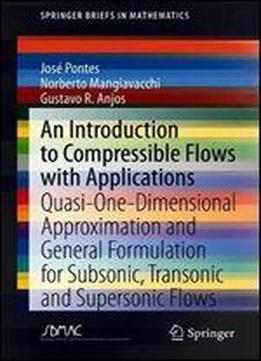# An Introduction To Compressible Flows With Applications: Quasi-one-dimensional Approximation And General Formulation For Subsonic, Transonic And Supersonic Flows (springerbriefs In Mathematics) by Jose Pontes / 2020 / English / PDF

This book offers a concise and practical survey of the principles governing compressible flows, along with selected applications. It starts with derivation of the time-dependent, three-dimensional equation of compressible potential flows, and a study of weak waves, including evaluation of the sound speed in gases. The following chapter addresses quasi-one-dimensional flows, the study of normal shock waves, and flow in ducts with constant cross section subjected to friction and/or heat transfer. It also investigates the effects of friction and heat transfer in ducts with variable cross section. The chapter ends by pointing to the analogy between one-dimensional compressible flows and open channel hydraulics. Further, the book discusses supersonic flows, including the study of oblique shock waves, and supersonic flows over corners and wedges. It also examines Riemann problems, numerical resolution of the wave equation, and of nonlinear hyperbolic problems, including propagation of strong waves. A subsequent chapter focuses on the small perturbation theory of subsonic, transonic and supersonic flows around slender bodies aligned or almost aligned to the uniform inflow. In particular, it explores subsonic and supersonic flows over a wavy wall. Lastly, an appendix with a short derivation of the Fluid Mechanics basic equations is included. The final chapter addresses the problem of transonic flows where both subsonic and supersonicare present. Lastly, an appendix with a short derivation of the Fluid Mechanics basic equations is included. Illustrated with several practical examples, this book is a valuable tool to understand the most fundamental mathematical principles of compressible flows. Graduate Mathematics, Physics and Engineering students as well as researchers with an interest in the aerospace sciences benefit from this work.

views: 136